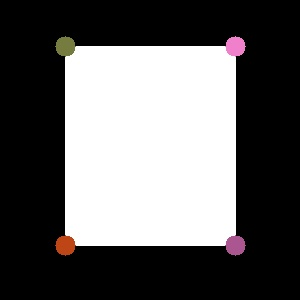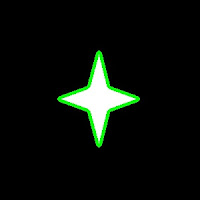close

# OpenCV-Python

### OpenCV Python Tutorials

opencvpython.blogspot.com

## Contours - 3 : Extraction

Hi,

This is our third article on contours and direct continuation of Contours 1 : Getting Started and Contours - 2 : Brotherhood. Hope you have read and understood it well before reading this.

In this article, we won't be using any new function from OpenCV, instead we use the methods from previous article to extract useful data of a contour or an object. You will be using some of these routines in your codes often. So we can get into the topic now.

What are these features actually ? Yes, that is a relative question, i think. It can be anything you want to find about an object and it directly depends on your goals. Some times, you may be interested in its size, sometimes its center, or its average color, or minimum and maximum intensity of that object, and even its orientation, ie its slope etc. I would like to list some of the normally used features.

1 - Area and Perimeter :

This, we have already discussed in last articles, which can be found out using cv2.contourArea() and cv2.arcLength() functions, respectively. You can refer that.

2 - Centroid :

Centroids are found using cv2.Moments() function where centroid can be defined as :

centroid_x = M10/M00 and centroid_y = M01/M00

`M = cv2.moments(cnt)centroid_x = int(M['m10']/M['m00'])centroid_y = int(M['m01']/M['m00'])`Remember, actual result obtained will be 'float', so convert it into 'int'.

If you draw a circle at that point, you can see the centroid.

3 - Aspect Ratio :

Aspect Ratio is the ratio of width to height.

It will be useful in the cases where you want to filter out some shapes. The best example which comes to my mind is ANPR (Automatic Number Plate Recognition). ANPR is used in several traffic surveillance systems to track vehicles going that way. So, in such scenarios, first step is to extract rectangles in the image (since number plate is a rectangle). But there may be false ones also. So use aspect ratio to remove unwanted rectangles (You can google several papers using this method)

`x,y,w,h = cv2.boundingRect(cnt)aspect_ratio = float(w)/h`

4 - Extent :

Extent is the ratio of contour area to bounding rectangle area.

`area = cv2.contourArea(cnt)x,y,w,h = cv2.boundingRect(cnt)rect_area = w*hextent = float(area)/rect_area`

5 - Solidity :

Solidity is the ratio of contour area to its convex hull area.

`area = cv2.contourArea(cnt)hull = cv2.convexHull(cnt)hull_area = cv2.contourArea(hull)solidity = float(area)/hull_area`

6 - Equivalent Diameter :

Equivalent Diameter is the diameter of the circle whose area is same as the contour area.

It is calculated as, Equivalent Diameter =  4 * A / Π
where A = Area of contour

`area = cv2.contourArea(cnt)equi_diameter = np.sqrt(4*area/np.pi)`

7 - Orientation :

Orientation is the angle at which object is directed.

`(x,y),(MA,ma),angle = cv2.fitEllipse(cnt)`

8 - Pixel Points :

In some cases, we may need all the points which comprises that object. It can be done as follows:

`mask = np.zeros(imgray.shape,np.uint8)cv2.drawContours(mask,[cnt],0,255,-1)pixelpoints = np.transpose(np.nonzero(mask))`

9 - Maximum Value and Minimum Value :

We can find these parameters using a mask image.

`min_val, max_val, min_loc,max_loc = cv2.minMaxLoc(imgray,mask = mask)`

where mask is same as above. Remember, this is for grayscale images, not for color images.

10 - Mean Color or Mean Intensity :

Here, we can find the average color of an object. Or it can be average intensity of the object in grayscale mode. We again use the same mask to do it.

`mean_val = cv2.mean(im,mask = mask)`

Remember, if you are trying for color matching or color based object tracking, first convert image to HSV space, because HSV is more better representation of color that RGB space. We will deal it in more detail in another article.

11 - Extreme Points :

Extreme Points means topmost,bottommost,rightmost,leftmost points of the object.

`leftmost = tuple(cnt[cnt[:,:,0].argmin()])rightmost = tuple(cnt[cnt[:,:,0].argmax()])topmost = tuple(cnt[cnt[:,:,1].argmin()])bottommost = tuple(cnt[cnt[:,:,1].argmax()])`

For eg, if I apply it to an Indian map, I get the following result :Extreme Points

For those who couldn't understand above piece of code, I will explain one for you, ie leftmost.

We have contour points (x,y) stored as a [rows,1,2]. Number of rows equal to number of contour points. So to find the leftmost point, we need to find the point where 'x' is minimum. 'y' doesn't matter. So for that, we extract 'x' coordinates of all the points.

`x = cnt[ : , : , 0]`

Now we find the location of minimum value in it.( Not minimum value, but position or index of the minimum value)

`x_min_loc = x.argmin()`

Now we find the point (x,y) in cnt at this location(x_min_loc).

`point = cnt[x_min_loc]`

Sometimes, there may be more than one leftmost points, like rectangles. So we have to take only one of them. And convert it into tuple.

`leftmost = tuple(point)`

So these are some of the features used frequently.

Now only few more things are there to explain about the contours like convexity defects, point polygon test etc. We will be dealing it in next article.

Regards,
ARK

## Contours - 1 : Getting Started

Hi, this article is a tutorial which try to cover all relevant functions in OpenCV dealing with Structural Analysis and Shape Descriptors, which are mainly related to contours.Contours can be explained simply as a curve joining all the continuous points (along the boundary), having same color or intensity. For example, consider image at left.

Assuming it is a binary image,we can say, its contour is the curve joining the all the boundary white points.

So if we find a contour in a binary image, we are finding the boundaries of objects in an image. That is why, OpenCV doc says, "The contours are a useful tool for shape analysis and object detection and recognition".

Finding Contours:

We start with a simple image as above. First we find the contours.

`import numpy as npimport cv2im = cv2.imread('test.jpg')imgray = cv2.cvtColor(im,cv2.COLOR_BGR2GRAY)ret,thresh = cv2.threshold(imgray,127,255,0)contours, hierarchy = cv2.findContours(thresh,cv2.RETR_TREE,cv2.CHAIN_APPROX_SIMPLE)`

Points to remember :
1. For better accuracy, use binary images. So before finding contours, apply threshold or canny edge detection.
2. FindContours function modifies the source image, so 'thresh' before and after finding contours are different image. So if you want 'thresh' as such after finding contours, store it to some other variables.
3. In OpenCV, its operation is like finding white object from black background. So remember, object to be found should be white and background in black.
What is structure of resulting contours?

The result "contours" is a Python list, where it contains all objects boundary points as separate lists. So to find number of objects, find length of list "contours", where in this case, it is one. Only one object. So we take it as "cnt".

`>>> len(contours)1>>> cnt = contours>>> len(cnt)244`

Here, number of points in cnt is 244. What these points denote? They are the boundary points of the object.

But, does it include all the boundary? Not exactly. The points are selected such that, contours can be drawn as straight line joining these points. So, if object is a horizontal or vertical line, only end points are stored. ie length of cnt = 2. If object is a rectangle, only 4 vertices are stored.Contour points for a rectangle

Thus in our image, there are no direct horizontal or vertical lines. So most of the points will be stored. To visualise it as above, you can draw circles for each value in cnt.

How to draw the contours?

For this, there is a function, cv2.drawContours(). Let's try it:

`cv2.drawContours(im,contours,-1,(0,255,0),3)`

This draws a 3-pixel wide green outline of the object. If you want to fill the object with a particular color, pass value of -1 to line thickness.

`cv2.drawContours(im,contours,-1,(0,255,0),-1)`Contours drawn filledContours drawn 3 px wide

Also, the third argument in cv2.drawContours() is also to be noted. Suppose, you want to draw only fourth contour(not here), third argument should be set to 3. If it is -1, all contours are drawn.

Now you want to draw "cnt" only. It can be done as follows:

`cv2.drawContours(im,[cnt],0,(255,0,0),-1)`

Note the square bracket around "cnt". Third argument set to 0, means only that particular contour is drawn.

Now we end after one more important concept, called Mask.

Mask can be considered as a binary image where only our desired area is white and all others are blacked out. They are used to isolate a part of image and do operations on that part only without affecting or operating on other parts of the image. This can also be considered as a ROI (Region of Interest) which can have any shape.Consider a scenario, where you are asked to find average colors of each shapes in the image at right. So simply threshold the image to binarize it (please don't ask me if white ball can be detected using thresholding, it is just an example). Find contours in the binary image, then for each contour, create a mask image of that shape. ie, if first ball is cosidered, the region of that ball in mask image will be white, while all other shapes and backgrounds are blacked out. Now if you can find the mean color of that shape only. So for every shapes.

(OK, just for this case, I will do it in this image, not on our original image at the beginning)

First we find the contours as we did before. (Adjust the threshold value to detect all). Now we will see how to do it:

First create a mask image where all elements are zero (ie just a black image) with size same as source, but single channel (ie grayscale).

Then for each contour, we draw it on the mask image filled with white color. Then we find mean using mean() function, taking our mask as operating mask.

`for h,cnt in enumerate(contours):    mask = np.zeros(imgray.shape,np.uint8)    cv2.drawContours(mask,[cnt],0,255,-1)    mean = cv2.mean(im,mask = mask)`Mask Images

See the result at left side.

(All the resulting images are animated to a single image)

I think it is sufficient for now. Keep these three in mind, ie Find Contours, Draw Contours and Mask Image. Now we can find some contour features in next post.

Regards,
ARK

## Report "OpenCV-Python"

Are you sure you want to report this post for ?

Cancel
×